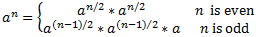## Problem

Need to implement a function which returns the n-th number in Fibonacci sequences with an input n. Fibonacci sequence is defined as:

```Works for n>=0;
f(0) = 0;
f(1) = 1;
for n>1  f(n)=f(n-2)+f(n-1);
```

## Solution 1 - bad solution

Looks good, but so slow.

```public int getFibbonacci(int n){
if (n<0) throw IllegalArgumentException("Illegal argument");
if (n==0) return 0;
if (n==1) return 1;
return getFibbonacci(n-1)+getFibbonacci(n-2);
}
```

Timing: You may not see result for n=O(n!) long time: n! time, Memory O(1) - no additional memory we need for variables, but we need a lot of memory for stack (for saving status for each invoke of function)

## Solution 2 - better solution - O(N)

```public int getFibbonacci(int n){
if (n<0) throw IllegalArgumentException("Illegal argument");
if (n==0) return 0;
if (n==1) return 1;       int prev=1;        int prevPrev =0;       int current;       for (int=2; i<n+1; i++){            current =  prev +prevPrev;            prevPrev = prev;            prev = current;       }
return current;
}
```

Timing: You may not see result for n=O(n!) time, Memory O(3) - no additional memory we need for variables (only 3 int variables)

## Solution 3 - O(logN)

Source

Usually interviewers expect the O(n) solution above. However, there is an O(logn) solution available, which is based on an uncommon equation as shown below:It is not difficult to prove this equation vithmathematical induction. Interested readers may have try.

Now the only problem is how to calculate power of a matrix. We can calculate power with exponent n in O(logn) time with the following equation:```#include

struct Matrix2By2
{
Matrix2By2
(
long long m00 = 0,
long long m01 = 0,
long long m10 = 0,
long long m11 = 0
)
:m_00(m00), m_01(m01), m_10(m10), m_11(m11)
{
}

long long m_00;
long long m_01;
long long m_10;
long long m_11;
};

Matrix2By2 MatrixMultiply
(
const Matrix2By2& matrix1,
const Matrix2By2& matrix2
)
{
return Matrix2By2(
matrix1.m_00 * matrix2.m_00 + matrix1.m_01 * matrix2.m_10,
matrix1.m_00 * matrix2.m_01 + matrix1.m_01 * matrix2.m_11,
matrix1.m_10 * matrix2.m_00 + matrix1.m_11 * matrix2.m_10,
matrix1.m_10 * matrix2.m_01 + matrix1.m_11 * matrix2.m_11);
}

Matrix2By2 MatrixPower(unsigned int n)
{
assert(n > 0);

Matrix2By2 matrix;
if(n == 1)
{
matrix = Matrix2By2(1, 1, 1, 0);
}
else if(n % 2 == 0)
{
matrix = MatrixPower(n / 2);
matrix = MatrixMultiply(matrix, matrix);
}
else if(n % 2 == 1)
{
matrix = MatrixPower((n - 1) / 2);
matrix = MatrixMultiply(matrix, matrix);
matrix = MatrixMultiply(matrix, Matrix2By2(1, 1, 1, 0));
}

return matrix;
}

long long Fibonacci(unsigned int n)
{
int result = {0, 1};
if(n < 2)
return result[n];

Matrix2By2 PowerNMinus2 = MatrixPower(n - 1);
return PowerNMinus2.m_00;
}
```

Even though it cost only O(logN) time in theory, its hidden constant parameter is quite big, so it is not treated as a practical solution in real software development. Additionally, it is not a recommended solution during interviews since its implementation code is very complex.